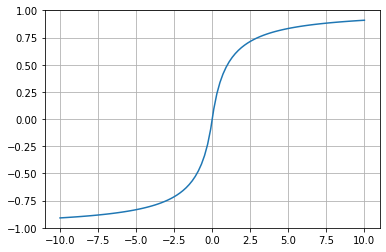## 常见激活函数 Activation Function¶

### 1. 激活函数概览¶

#### 1.1 什么是激活函数¶### 2. 常见的激活函数¶

#### 2.1 Sigmoid¶

Sigmoid函数是最基础也是曾经被应用最多的激活函数，它被广泛应用到BP神经网络，最初的ANN和MLP中。直到今天，在诸如循环神经网络RNN, 概率模型, 自编码器AutoEncode中仍然被广泛使用。根据它的形状，我们有时候也称它为S形函数。下面给出Sigmoid函数的数学表达Python Code.

$$f(x) = \frac{1}{1+e^{-x}}$$
In :
import numpy as np
import matplotlib.pyplot as plt

def sigmoid(x):
y=1.0/(1.0+np.exp(-x))
return y

x=np.linspace(start=-10,stop=10,num=100)
y=sigmoid(x)

plt.plot(x,y)
plt.grid(True)
plt.show()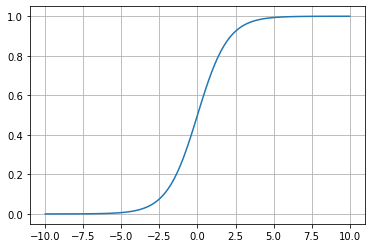#### 2.2 tanh¶

$$f(x) = \frac{1-e^{-2x}}{1+e^{-2x}}$$
In :
import numpy as np
import matplotlib.pyplot as plt

def tanh(x):
y=(1.0-np.exp(-2*x))/(1.0+np.exp(-2*x))
return y

x=np.linspace(start=-10,stop=10,num=100)
y=tanh(x)

plt.plot(x,y)
plt.grid(True)
plt.show()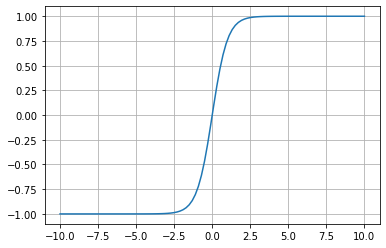#### 2.3 ReLU¶

ReLU是目前使用最广的激活函数，也是目前深度学习、卷积神经网络的事实标准激活函数，需要每个同学都认真掌握。

ReLU的数学表达式（简单之美）：

$$f(x) = max(0, x)$$

$$f(x) = \begin{cases} x & x \geq 0 \\ 0 & x < 0 \\ \end{cases}$$

ReLU的有效性体现在两个方面：

• 克服梯度消失的问题
• 加快训练速度

In :
import numpy as np
import matplotlib.pyplot as plt

def relu(x):
y=x.copy()
y[y<0]=0
return y

x=np.linspace(start=-10,stop=10,num=100)
y=relu(x)
# print(y)
plt.plot(x,y)
plt.grid(True)
plt.show()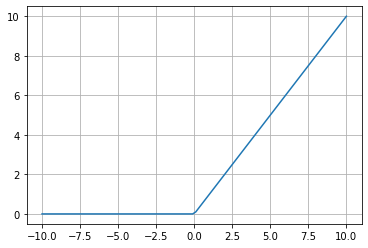#### 2.4 LReLU, PReLU, RReLU¶

$$f(y_i) = \begin{cases} y & x \geq 0 \\ a_i y_i & x < 0 \\ \end{cases}$$

• LReLU(Leaky ReLU), $a$ 是固定的, 需要通过各种验证方式选出一个合适的a值
• PReLU(Parametric ReLU), 对LReLU的改进, 可以自适应的学习参数而不是固定的, 原论文中建议初始化为0.25
• RReLU(Randomized Leaky ReLU), $a$ 是一个给定范围内的随机变量, 随机选择的, 在一定程度上可以起到正则化的作用In :
# ReLU
import numpy as np
import matplotlib.pyplot as plt

def lrelu(x,a):
y=x.copy()
for i in range(y.shape):
if y[i]<0:
y[i]=a*y[i]
return y

x=np.linspace(start=-10,stop=10,num=100)
y=lrelu(x,0.25)
# print(x)
# print(y)
plt.plot(x,y)
plt.grid(True)
plt.show()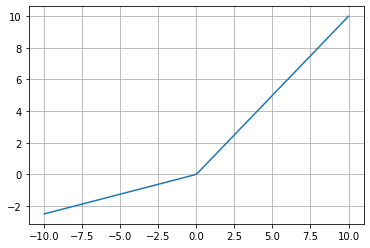#### 2.5 ELU¶

Exponential Linear Units的缩写,函数形式为

$$f(x) = \begin{cases} x & x \geq 0 \\ a(a^x - 1) & x < 0 \\ \end{cases}$$

In :
import numpy as np
import matplotlib.pyplot as plt

def elu(x,a):
y=x.copy()
for i in range(y.shape):
if y[i]<0:
y[i]=a*(np.exp(y[i])-1)
return y

x=np.linspace(start=-10,stop=10,num=100)
y=elu(x,0.5)
# print(x)
# print(y)
plt.plot(x,y)
plt.grid(True)
plt.show()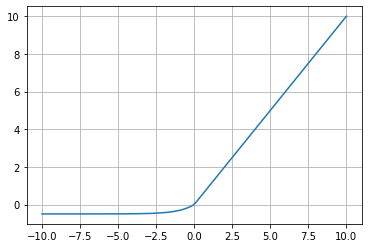#### 2.6 Softplus¶

$$f(x) = log(e^x + 1)$$
In :
import numpy as np
import matplotlib.pyplot as plt

def softplus(x):
y=np.log(np.exp(x)+1)
return y

x=np.linspace(start=-10,stop=10,num=100)
y=softplus(x)

plt.plot(x,y)
plt.grid(True)
plt.show()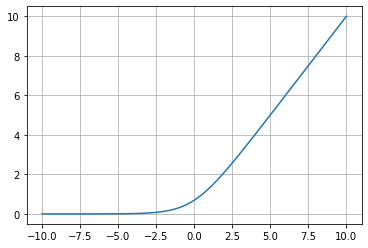#### 2.7 Softsign¶

$$f(x) = \frac{x}{|x| + 1}$$
In :
import numpy as np
import matplotlib.pyplot as plt

def softsign(x):
y=x/(np.abs(x)+1)
return y

x=np.linspace(start=-10,stop=10,num=100)
y=softsign(x)

plt.plot(x,y)
plt.grid(True)
plt.show()